# ISEE Middle Level Math : Data Analysis

## Example Questions

### Example Question #161 : Data Analysis

Find the mode in this set of numbers: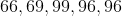Possible Answers: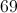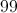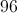Correct answer:Explanation:

The mode is the most recurring number:### Example Question #162 : Data Analysis

Find the mode in this set of numbers: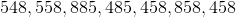Possible Answers: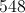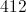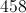Correct answer:Explanation:

Find the most recurring number: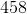### Example Question #41 : How To Find Mode

Find the mode of this set of numbers: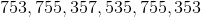Possible Answers: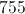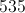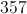Correct answer:Explanation:

Find the most recurring number: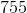### Example Question #42 : How To Find Mode

Find the mode of the set of numbers: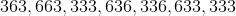Possible Answers: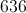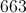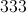Correct answer:Explanation:

The mode is the number that appears most frequently in the data set: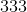### Example Question #43 : How To Find Mode

Find the mode in this set of numbers: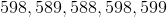Possible Answers: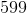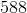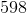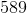Correct answer:Explanation:

Identify the most recurring number:Answer: The mode is 598.

### Example Question #44 : How To Find Mode

Find the mode in this set of numbers: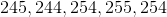Possible Answers: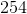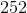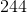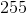Correct answer:Explanation:

Identify the most recurring number in the set:Answer: The mode is 254.

### Example Question #45 : How To Find Mode

Find the mode in this set of numbers: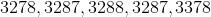Possible Answers: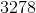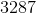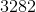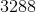Correct answer:Explanation:

Find the most recurring number in the set:### Example Question #46 : How To Find Mode

Find the mode in this set of numbers: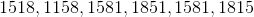Possible Answers: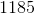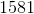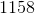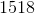Correct answer:Explanation:

Identify the number that appears most frequently:### Example Question #47 : How To Find Mode

Find the mode in this set of numbers: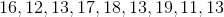Possible Answers:Correct answer:Explanation:

Find the most recurring number:Answer: The mode is.

### Example Question #48 : How To Find Mode

Find the mode in this set of numbers: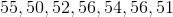Possible Answers:Correct answer:Explanation:

Find the most recurring number in this set:Answer: The mode is.

### All ISEE Middle Level Math Resources